### 16.11.6.Usage Statistics

1. Use the Faceted Browser Search and Find User Interface to search for information on "Michael Jackson":

Figure16.138.Usage Statistics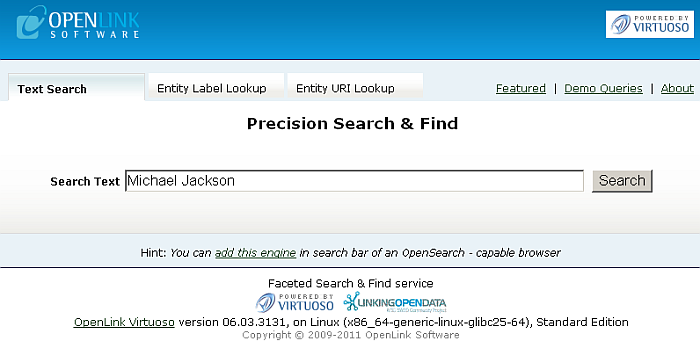2. Results of the following form should be returned for the network resource data being fetched.

Figure16.139.Usage Statistics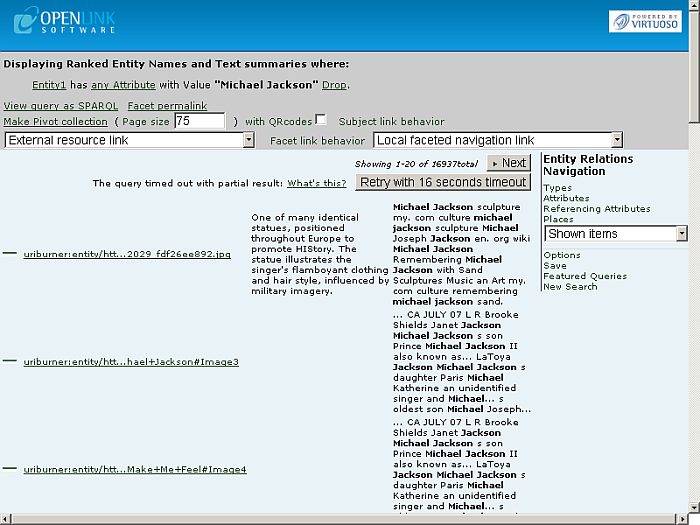3. Click the "Types" link under "Entity Relations Navigation".

4. Results about "Michael Jackson" as Type/Label/Count list should be displayed:

Figure16.140.Usage Statistics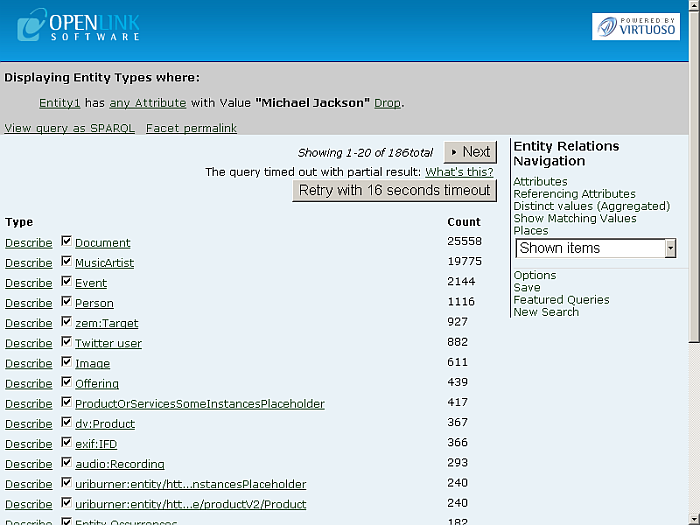5. You can navigate amongst the search results pages by using the "Prev" and "Next" buttons. Click for ex. "Next":

Figure16.141.Usage Statistics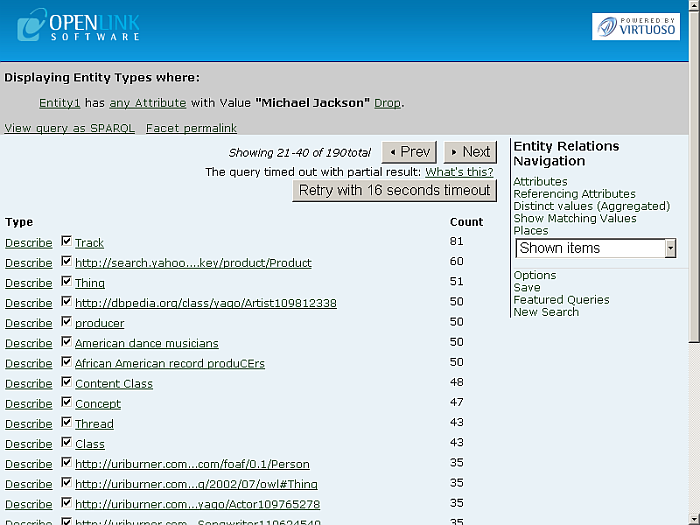6. Click a type link, for ex.:

```http://dbpedia.org/class/yago/Artist109812338
```
7. Should be shown type results and:

```Displaying Ranked Entity Names and Text summaries where:

Entity1 has any Attribute with Value "Michael Jackson" Drop.
Entity1 is a yago:Artist109812338 . Drop
```

Figure16.142.Usage Statistics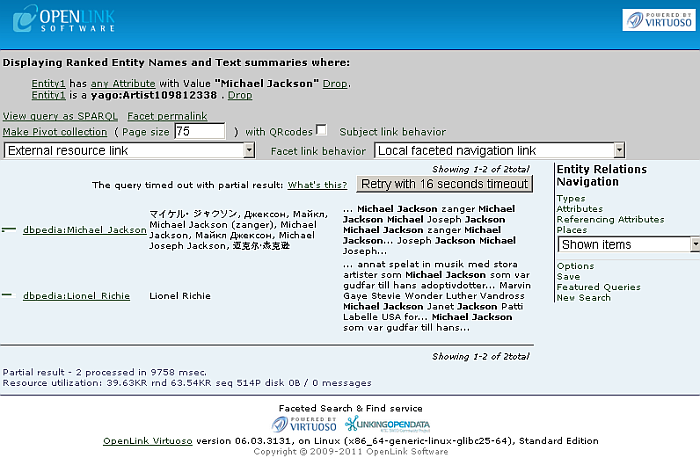8. Click the link:

```dbpedia:Michael_Jackson
```
9. Results about "Michael Jackson" as Attribute/Value list should be presented:

Figure16.143.Usage StatisticsFigure16.144.Usage Statistics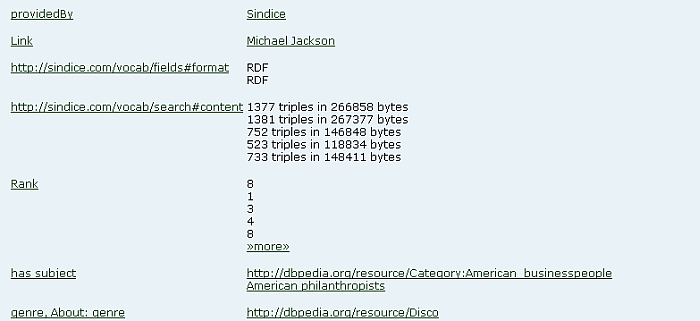Figure16.145.Usage StatisticsFigure16.146.Usage Statistics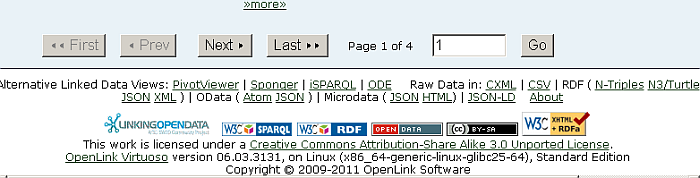10. You can navigate amongst the search results pages by using the "First", "Prev", "Next" and "Last" buttons. Click for ex. "Last":

Figure16.147.Usage StatisticsFigure16.148.Usage Statistics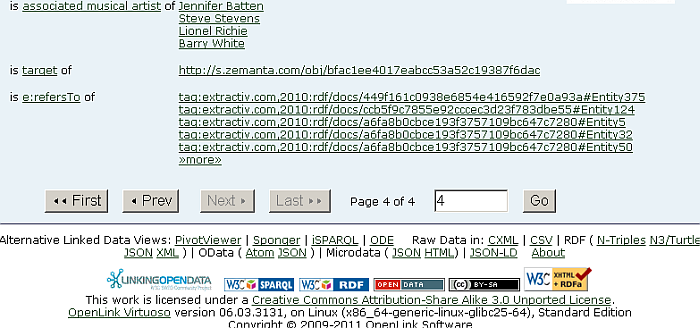12. Results of usage statistics for "Michael Jackson" grouped in 4 tabs should be shown:

1. Referenced by Graphs: shows how many times the URI is found as subject in the relevant graph(s):

```SPARQL
SELECT ?g count (*)
where
{
graph ?g { <URI> ?p ?o }
}
group by ?g
order by desc 2
limit 20
```

Figure16.149.Usage Statistics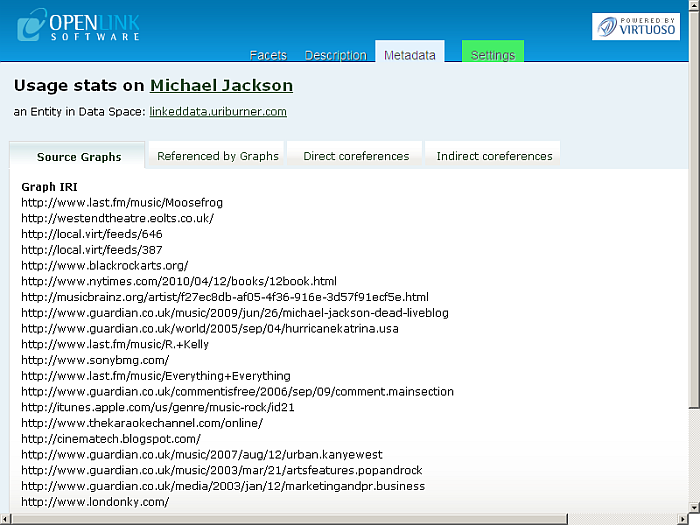2. Source Graphs: shows how many times the URI is found as object in the relevant graph(s):

```SPARQL
SELECT ?g count (*)
where
{
graph ?g { ?s ?p <URI>  }
}
group by ?g
order by desc 2
limit 20
```

Figure16.150.Usage Statistics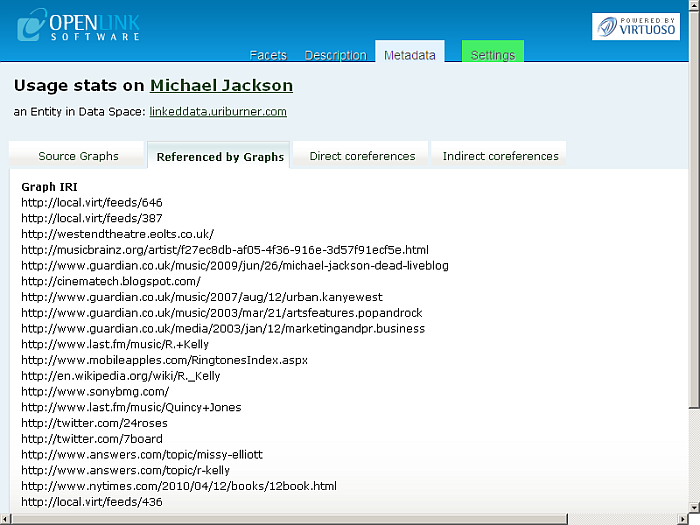3. Direct co-references: shows results as subject and calculated rank, based on running transitive closure over owl:sameAs of the URI in subject or object:

```SPARQL
SELECT ?syn ( sql:rnk_scale (<LONG::IRI_RANK> (?syn)))
where
{
{ SELECT ?s ?syn
where
{
{?syn owl:sameAs ?s } union {?s owl:sameAs ?syn}
}
}
option (transitive, t_distinct, t_min (0), T_in (?s), t_out (?syn)) . filter (!isliteral (?syn) &amp;&amp; ?s = <URI> )
}
order by desc 2
limit 20
```

Figure16.151.Usage Statistics4. Indirect co-references: shows expanded results for objects concur with the URI by IFP:

```SPARQL
SELECT distinct ?syn ?p ?o (sql:rnk_scale (<LONG::IRI_RANK> (?syn)))
where
{ <URI> ?p ?o .  filter (0 != (<LONG::bif:rdf_is_sub> ("b3sifp", ?p, lod:ifp_like, 3))) .
?syn ?p ?o .
}
order by desc 4
limit 20
```

Figure16.152.Usage Statistics